﻿ Calculate prices automatically
ingenious
Calculate prices automatically
 The main modules > Articles > Article detail view > Calculate prices automatically

For scaled prices and price matrices a function for automatic price calculation is available.

Price matrices are prices tables, with that prices per measurements (width/height) can be calculated. Diverse price lists can be created as a base for the calculation of project items in project documents or for other calculations.

For each price matrix a single article is created in the product catalogue. In the column prices the related price type and scale is to be chosen. For a 2-dimensional price matrix (Scale X/Y) a table sheet is provided with the scale values for width and height in its axes. These values can be automatically filled by entering the first value, the interval and the last value (e.g. 100/20/300) in the first grey field.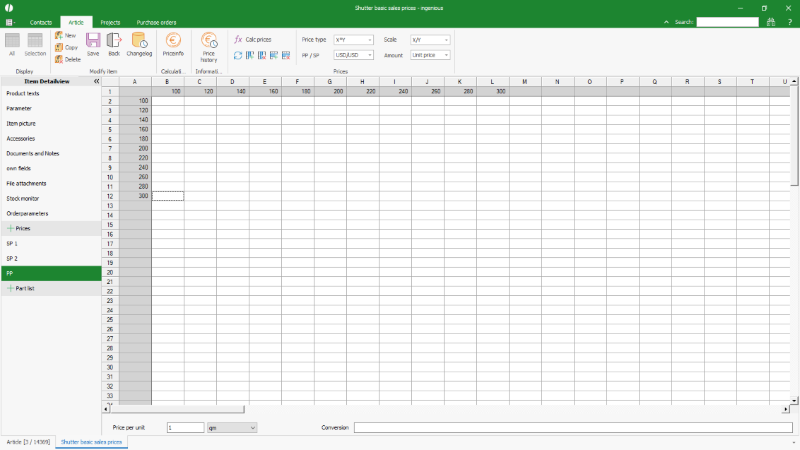Labeling of the axes of a price matrix

Also the prices themselves can be automatically filled. With the button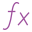and its drop down menue divers functions are available.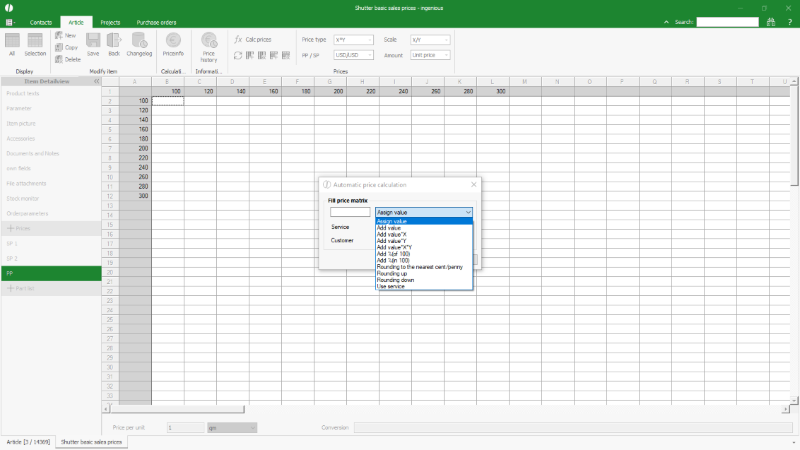Functions to calculate a price matrix

#### Calculate prices by an existing part list

A price matrix can be filled by means of a part list (service). By that way price lists can be calculated e.g. from the purchase price of all in a part list used single items and their quantities to then calculate the sales prices with a percental surcharge.

For this calculation first the option "Use service" has to be chosen from drop down menue. Afterwards the base for the price calculation has to be defined (PP, SP1 or SP2). In the field "service" the matchcode of the part list has to be entered. If the price matrix shall be calculated for a customer with his individual special prices, also his matchcode has to be entered in the related field.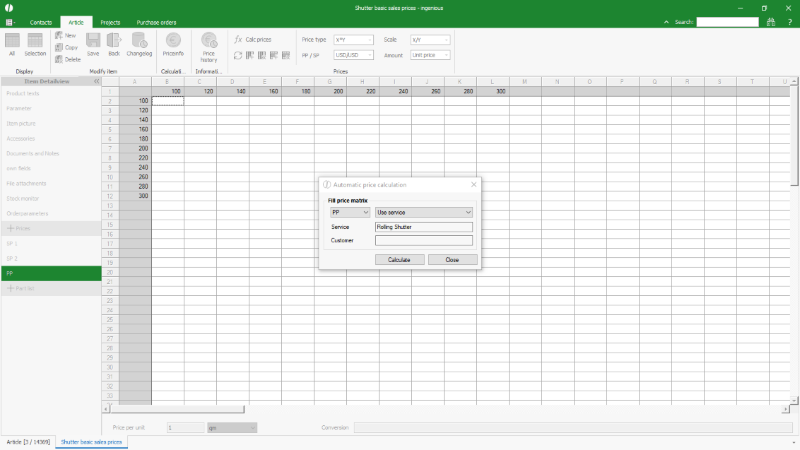Calculating a price matrix with a part list

After clicking "Calculate" the configurator is opened, with that the options for the variant, that needs to be calculated, can be chosen.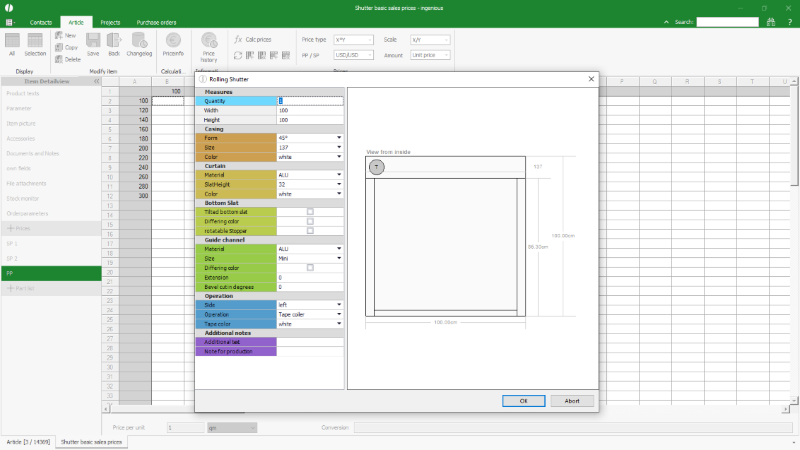Configuration of the part list options for the price matrix

After the confirmation of the configuration the price matrix is filled. If the calculation causes errors, e.g. because of the technical dependencies that are defined in the part list, the further calculation can be canceled or continued. The continued calculation after a planned error produces a price of "0" for the technical impossible measurements. This zero-price itself reflects the technical restriction.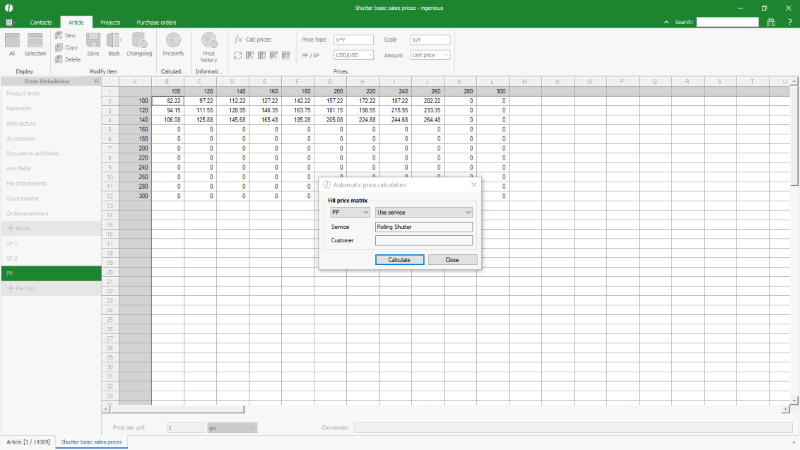With a part list calculated price matrix

In the still opened price calculation dialogue additional modifications of the prices can be made, e.g. add a surcharge of 50% and round the prices to full Euros/Dollars/Pounds.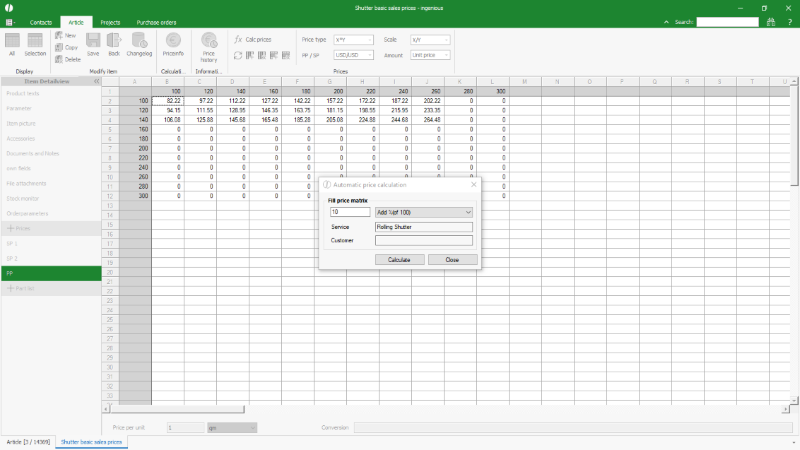Further price modification of a price matrix

The intermediate steps of the price changes are executed immediately and the results can directly be followed in the price list.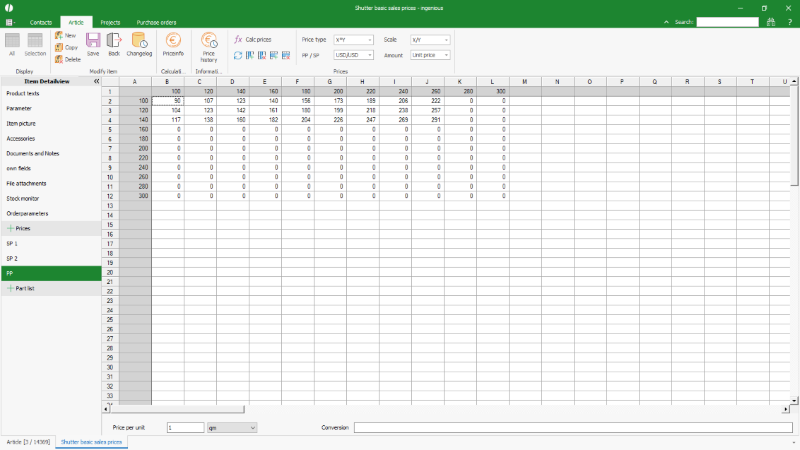Finalised price matrix